• 前缀和
千次阅读
2022-03-09 18:21:27

Python itertools accumulate

# 差分 Difference

数组 [1,2,2,4]，其差分数组为 [1,1,0,2]，差分数组的第 i 个数即为原数组的第 i 个元素和第 i -1 个元素的差值，对差分数组求前缀和即可得到原数组。

arr = [1,2,2,4]
d =  * len(arr) # 差分数组
d = arr
for i in range(1,len(arr):
d[i] = arr[i] - arr[i-1]


差分数组 d，d[i] += x，相当于原数组从第 i 项开始每个元素加 x。
当对原数组的某一个区间 [l, r] 施加一个增量 inc 时，d 对应的改变是：d[l] += inc，d[r+1] -= inc。对区间的修改转变为对两个位置的修改

# 1109.航班预订统计

class Solution:
def corpFlightBookings(self, bookings: List[List[int]], n: int) -> List[int]:
res =  * n
for f, t, s in bookings: # 从 f 到 t 各个航班
res[f-1] += x # 从 f-1 (航班转换为索引) 开始 +
if t < n:
res[t] -= x # 到 t-1 后面结束 -。

return list(accumulate(res)) # 前缀和统计

class Solution {
public int[] corpFlightBookings(int[][] bookings, int n) {
int[] res = new int[n];
for (int[] b : bookings){
res[b - 1] += b;
if (b < n) res[b] -= b;
}
for (int i = 1; i < n; i++) res[i] += res[i-1];
return res;
}
}


# 1854.人口最多的年份

class Solution:
def maximumPopulation(self, logs: List[List[int]]) -> int:
# d = {i:0 for i in range(1950,2051)}
# # d = defaultdict(int) 得到的可能不是最早的年份
# for x, y in logs:
#     for i in range(x, y): # 活着的区间 对人数贡献为 1
#         d[i] += 1

# res, year = 0, 0
# for i in d: # 找人数最多的年份
#     if d[i] > res:
#         res = d[i]
#         year = i

# return year

delta =  * 101 # 变化量 出生 - 死亡
offset = 1950 # 起始年份与起始下标之差 年份需要映射到列表
for i, j in logs:
delta[i - offset] += 1
delta[j - offset] -= 1
mx = res = cur = 0   # 人数最大值，对应的最小下标，每一年的人数
# 前缀和
for i in range(101):
cur += delta[i] # 前缀和
if cur > mx:
mx = cur
res = i
return res + offset   # 转回对应的年份


# 798.得分最高的最小轮调

nums = [2,3,1,4,0]，值 ≤ \le 下标 得分，
2，1 ~ 3 步；diff[0+1]++, diff[0+5-2+1]–
3，2 ~ 3 步；diff[1+1]++, diff[1+5-3+1]–
1，分两段，0 ~ 1 步。第 2 步不得分，3 ~ 4 步；diff++, diff[(2+5-1+1)%5]–,diff[2+1]++
4，4 步；diff[3+1]++
0，0 ~ 4 步。diff ++

class Solution:
def bestRotation(self, nums: List[int]) -> int:
n = len(nums)
d =  * n
for i, x in enumerate(nums):
if x <= i: d += 1 # 左移 0 ~ i-x 步得分
d[(i-x+1+n)%n] -= 1 # 当前得分情况下左移 i-x+1 步，否则 先左移 i 步，右移，再左移 n-x+1 步，终止得分。
d[(i+1)%n] += 1 # 右移开始得分

score = maxScore = idx = 0
for i, diff in enumerate(d):
score += diff
if score > maxScore:
maxScore, idx = score, i
return idx


# 1310.子数组异或查询

class NumArray:

def __init__(self, nums: List[int]):
self.nums = nums
self.acc = list(accumulate(nums))

def sumRange(self, left: int, right: int) -> int:
return self.acc[right] - (self.acc[left-1] if left else 0)


# 6158. 字母移位 II

class Solution:
def shiftingLetters(self, s: str, shifts: List[List[int]]) -> str:
n = len(s)
q =  * (n + 1)
for i, j, k in shifts:
k = 2 * k - 1
q[i] += k
q[j+1] -= k
acc = list(accumulate(q[:-1]))

return ''.join(chr((x + ord(c) - ord('a')) % 26 + ord('a')) for x, c in zip(acc, s))


# 前缀和 Prefix sum

itertools.accumulate(iterable[, func, *, initial=None])
accumulate([1,2,3,4,5]) --> 1 3 6 10 15
accumulate([1,2,3,4,5], initial=100) --> 100 101 103 106 110 115
accumulate([1,2,3,4,5], operator.mul) --> 1 2 6 24 120


# 724.寻找数组的中心下标

class Solution:
def pivotIndex(self, nums: List[int]) -> int:
pre, cur, s = 0, 0, sum(nums)
for i, x in enumerate(nums):
cur += x
if s - cur == pre: return i
pre = cur
return -1

class Solution {
public int pivotIndex(int[] nums) {
int sum = 0, pre = 0, cur = 0;
for (int x : nums) sum += x;
for (int i = 0; i < nums.length; i++) {
cur += nums[i];
if (sum - cur == pre) return i;
pre = cur;
}
return -1;
}
}


# 1.两数之和

class Solution:
def twoSum(self, nums: List[int], target: int) -> List[int]:
d = {}
for i, x in enumerate(nums):
if target - x in d: return [d[target - x], i]
d[x] = i
return []


# 560.和为K的子数组

class Solution:
def subarraySum(self, nums: List[int], k: int) -> int:
res, presum, d = 0, 0, defaultdict(int)
for x in nums:
d[presum] += 1
presum += x
res += d[presum - k]
return res

class Solution {
public int subarraySum(int[] nums, int k) {
int res = 0, presum = 0;
Map<Integer, Integer> map = new HashMap<>();
for (int x : nums){
map.put(presum, map.getOrDefault(presum, 0) + 1);
presum += x;
res += map.getOrDefault(presum - k, 0);
}
return res;
}
}


# 930.和相同的二元子数组

前缀和数组 preSum，子数组 (i,j] 的和为 goal，即：preSum[j] − preSum[i] = goal。枚举 j ，每次查询满足该等式的 i 的数量。

用哈希表记录每一种前缀和出现的次数，当前枚举到元素 nums[j]，查询哈希表中元素 preSum[j] − goal，即对应了以当前 j 值为右边界的满足条件的子数组的数量。最后这些元素的总数量即为所有和为 goal 的子数组数量。

实时地更新哈希表，以防止出现 i ≥ j 的情况。

class Solution:
def numSubarraysWithSum(self, nums: List[int], goal: int) -> int:
res = preSum = 0 # 计算前缀和，不用实现前缀和数组
d = defaultdict(int) # 统计出现前缀和 preSum 的个数
for x in nums:
d[preSum] += 1
preSum += x
res += d[preSum - goal]
return res

class Solution {
public int numSubarraysWithSum(int[] nums, int goal) {
int res = 0, presum = 0;
int[] d = new int[nums.length + 1];
for (int x : nums){
d[presum] += 1;
presum += x;
res += (presum >= goal ? d[presum - goal] : 0);
}
return res;
}
}


# 1248.统计「优美子数组」

d 的 key 是前缀和（即当前奇数的个数），value 是前缀和的个数。可以用数组。
遍历原数组，计算当前的前缀和，统计到 d 中，并且在 res 中累加上与当前前缀和差值为 k 的前缀和的个数。

class Solution:
def numberOfSubarrays(self, nums: List[int], k: int) -> int:
res, presum, d = 0, 0, defaultdict(int)
for x nums:
d[presum] += 1
presum += x & 1 # Prefix sum 即当前奇数的个数
if presum >= k: res += d[presum - k]

return res

class Solution {
public int numberOfSubarrays(int[] nums, int k) {
int[] precnt = new int[nums.length + 1];
int res = 0, presum = 0;
for (int x: nums) {
precnt[presum]++;
presum += x & 1;
if (presum >= k) res += precnt[presum - k];
}
return res;
}
}


# 974.和可被K整除的子数组

同余定理：(a - b) % k = 0 等价于 a % k = b % k

class Solution:
def subarraysDivByK(self, nums: List[int], k: int) -> int:
res, presum, d = 0, 0, defaultdict(int) # 保存余数
for x in nums:
d[presum % k] += 1
presum += x
res += d[presum % k]
return res

class Solution {
public int subarraysDivByK(int[] nums, int k) {
int res = 0, presum = 0;
int[] d = new int[k];
d = 1;
for (int x : nums){
presum += x;
res += d[(presum % k + k) % k]++;
}
return res;
}
}


# 523.连续的子数组和

同余定理 a ≡ b(mod d) 即 d 整除 a - b

class Solution:
def checkSubarraySum(self, nums: List[int], k: int) -> bool:
presum, d = 0, {0:-1} # 哨兵
for i, x in enumerate(nums):
presum += x
key = presum % k
if key in d:
if i - d[key] >= 2: return True
else: d[key] = i  # 保存最小索引

return False

class Solution {
public boolean checkSubarraySum(int[] nums, int k) {
HashMap<Integer,Integer> map = new HashMap<>();
map.put(0,-1); // 哨兵
int presum = 0;
for (int i = 0; i < nums.length; ++i) {
presum += nums[i];
int key = presum % k;
if (map.containsKey(key)) {
if (i - map.get(key) >= 2) return true;
} else map.put(key, i);  // 保存最小索引
}
return false;
}
}


# ★862.和至少为K的最短子数组

综合题：前缀和、单调队列，滑动窗口。

class Solution:
def shortestSubarray(self, nums: List[int], k: int) -> int:
n = len(nums)
res, q = n + 1, collections.deque()
acc =  + list(accumulate(nums))
for i, x in enumerate(acc):
while q and x <= acc[q[-1]]: q.pop() # 单调队列
while q and x - acc[q] >= k:
res = min(res, i - q.popleft())
q.append(i) # 下标
return res if res <= n else -1

class Solution {
public int shortestSubarray(int[] nums, int k) {
int n = nums.length;
int ans = n + 1;
long[] acc = new long[n + 1];
Deque<Integer> q = new LinkedList();
for (int i = 0; i < n; i++) acc[i + 1] = acc[i] + (long) nums[i];
for (int i = 0; i < acc.length; i++){
while (!q.isEmpty() && acc[i] <= acc[q.getLast()]) q.removeLast();
while (!q.isEmpty() && acc[i] >= acc[q.getFirst()] + k) ans = Math.min(ans, i - q.removeFirst());
}
return ans <= n ? ans : -1;
}
}leetcode
更多相关内容
• 前缀和： 前缀和是指某序列的前n项和，可以把它理解为数学上的数列的前n项和（对于一个一维数组的前缀和） ** ** 前缀和算法有什么好处？ 先来了解这样一个问题： 输入一个长度为n的整数序列。接下来再输入m个询问...

## 1、前缀和

前缀和是指某序列的前n项和，可以把它理解为数学上的数列的前n项和，而差分可以看成前缀和的逆运算。合理的使用前缀和与差分，可以将某些复杂的问题简单化。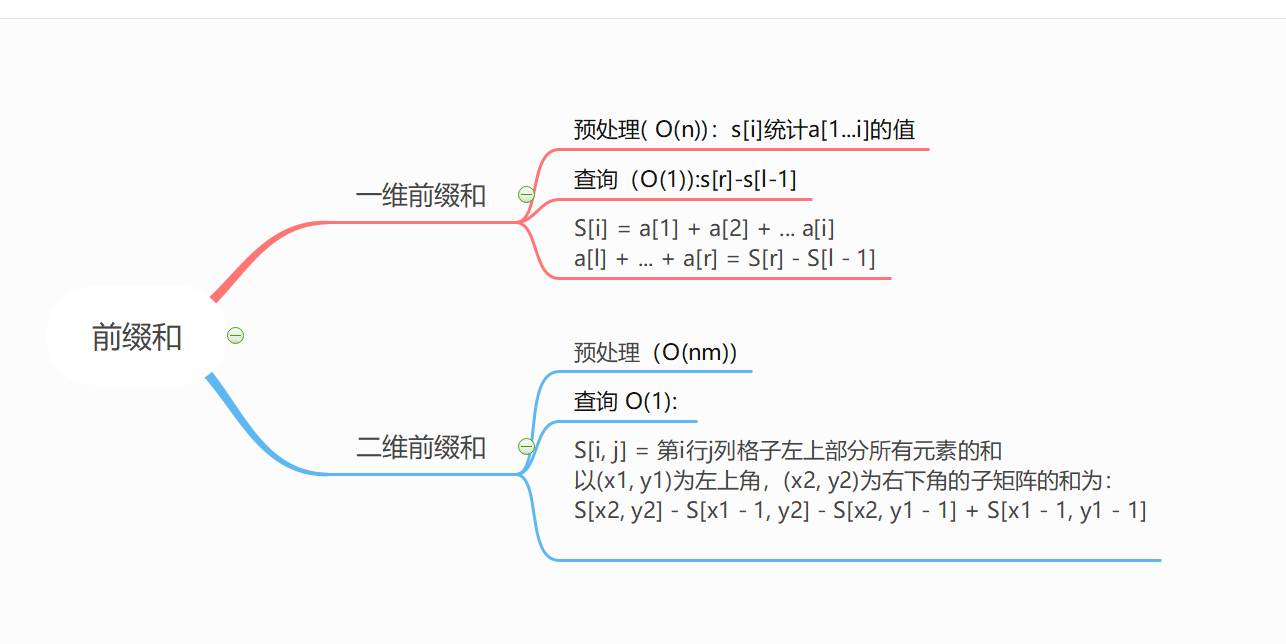## 2、前缀和算法有什么好处？

先来了解这样一个问题：

输入一个长度为n的整数序列。接下来再输入m个询问，每个询问输入一对l, r。对于每个询问，输出原序列中从第l个数到第r个数的和。

我们很容易想出暴力解法，遍历区间求和。

代码如下：

const int N = 1e5 + 10;
int a[N];
int n,m;
scanf("%d%d", &n, &m);
for(int i = 1; i <= n; i++) scanf("%d", &a[i]);
while(m--)
{
int l, r;
int sum = 0;
scanf("%d%d", &l, &r);
for(int i = l; i <= r; i++)
{
sum += a[i];
}
printf("%d\n",sum);
}


这样的时间复杂度为O(n * m)，如果nm的数据量稍微大一点就有可能超时，而我们如果使用前缀和的方法来做的话就能够将时间复杂度降到O(n + m)，大大提高了运算效率。

具体做法：

首先做一个预处理，定义一个sum[]数组，sum[i]代表a数组中前i个数的和。

求前缀和运算：

const int N = 1e5 + 10;
int sum[N], a[N]; //sum[i]=a+a+a.....a[i];
for(int i = 1;i <= n; i++)
{
sum[i] = sum[i - 1] + a[i];
}


然后查询操作：

 scanf("%d%d",&l,&r);
printf("%d\n", sum[r] - sum[l - 1]);


对于每次查询，只需执行sum[r] - sum[l - 1] ，时间复杂度为O(1)

原理

sum[r] = a + a + a + a[l-1] + a[l] + a[l + 1] ...... a[r];
sum[l - 1] = a + a + a + a[l - 1];
sum[r] - sum[l - 1] = a[l] + a[l + 1] + ......+ a[r];

图解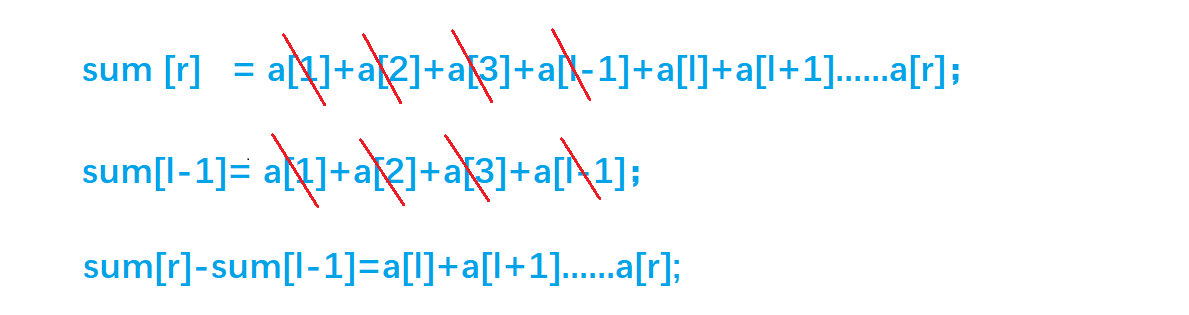这样，对于每个询问，只需要执行 sum[r] - sum[l - 1]。输出原序列中从第l个数到第r个数的和的时间复杂度变成了O(1)

我们把它叫做一维前缀和

总结：练习一道题目
输入一个长度为n的整数序列。
接下来再输入m个询问，每个询问输入一对l, r。
对于每个询问，输出原序列中从第l个数到第r个数的和。
输入格式
第一行包含两个整数n和m。
第二行包含n个整数，表示整数数列。
接下来m行，每行包含两个整数l和r，表示一个询问的区间范围。
输出格式
共m行，每行输出一个询问的结果。
数据范围
1≤l≤r≤n,
1≤n,m≤100000,
−1000≤数列中元素的值≤1000
输入样例：

5 3
2 1 3 6 4
1 2
1 3
2 4


输出样例：

3
6
10


代码：

#include <iostream>

using namespace std;

const int N = 100010;

int n, m;
int a[N], s[N];

int main()
{
scanf("%d%d", &n, &m);
for (int i = 1; i <= n; i ++ ) scanf("%d", &a[i]);

for (int i = 1; i <= n; i ++ ) s[i] = s[i - 1] + a[i]; // 前缀和的初始化

while (m -- )
{
int l, r;
scanf("%d%d", &l, &r);
printf("%d\n", s[r] - s[l - 1]); // 区间和的计算
}

return 0;
}


## 3、二维前缀和

如果数组变成了二维数组怎么办呢？

先给出问题：

输入一个nm列的整数矩阵，再输入q个询问，每个询问包含四个整数x1, y1, x2, y2，表示一个子矩阵的左上角坐标和右下角坐标。对于每个询问输出子矩阵中所有数的和。

同一维前缀和一样，我们先来定义一个二维数组s[][] , s[i][j] 表示二维数组中，左上角(1, 1)到右下角(i, j)所包围的矩阵元素的和。接下来推导二维前缀和的公式。

先看一张图：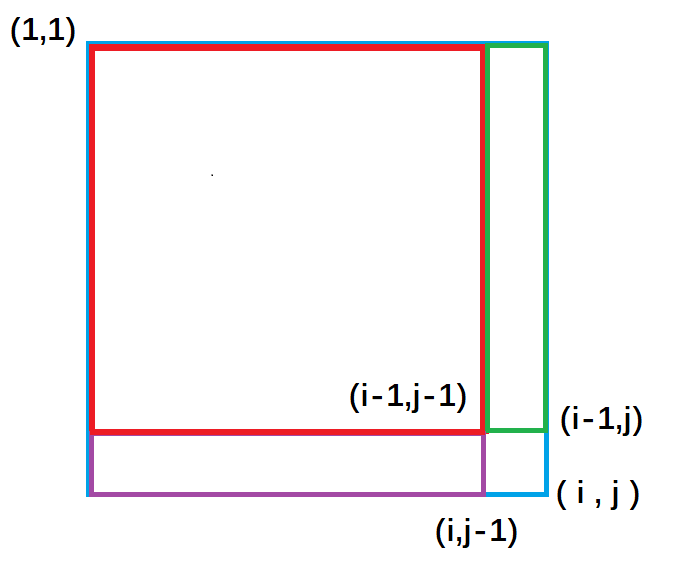紫色面积是指(1, 1)左上角到(i, j - 1)右下角的矩形面积, 绿色面积是指(1, 1)左上角到(i - 1, j )右下角的矩形面积。每一个颜色的矩形面积都代表了它所包围元素的和。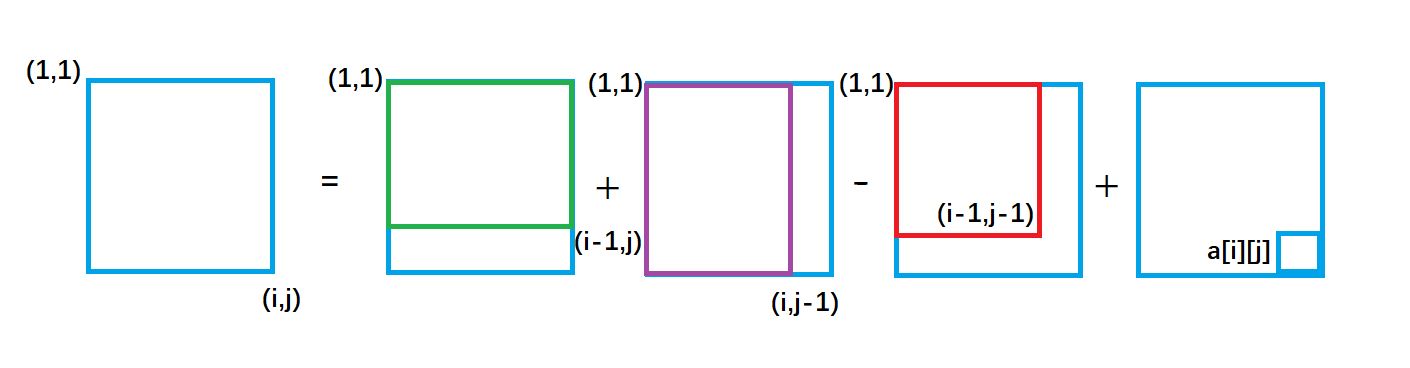从图中我们很容易看出，整个外围蓝色矩形面积s[i][j] = 绿色面积s[i - 1][j] + 紫色面积s[i][j - 1] - 重复加的红色的面积s[i - 1][j - 1] + 小方块的面积a[i][j];

因此得出二维前缀和预处理公式

s[i][j] = s[i - 1][j] + s[i][j - 1 ] + a[i] [j] - s[i - 1][j - 1]

接下来回归问题去求以(x1,y1)为左上角和以(x2,y2)为右下角的矩阵的元素的和。

如图：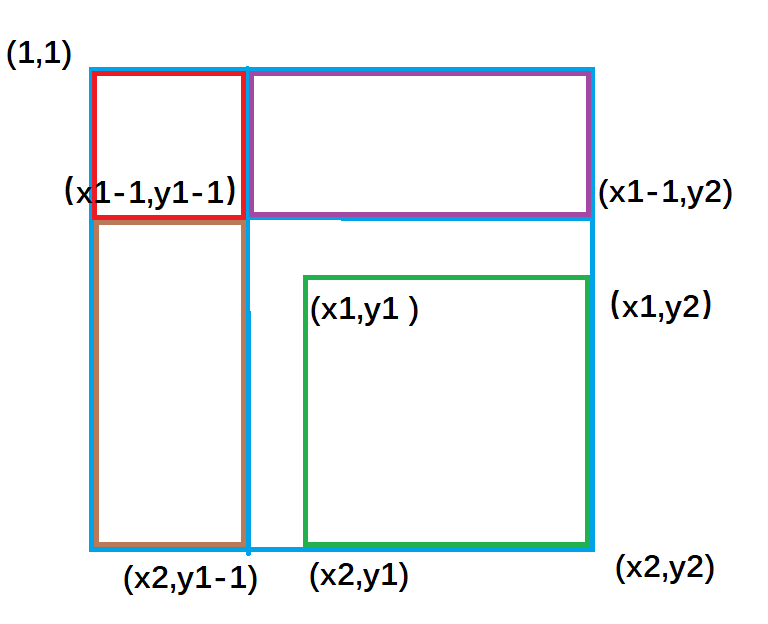紫色面积是指 (1, 1)左上角到(x1 - 1, y2)右下角的矩形面积 ，黄色面积是指(1, 1)左上角到(x2, y1 - 1)右下角的矩形面积；

不难推出：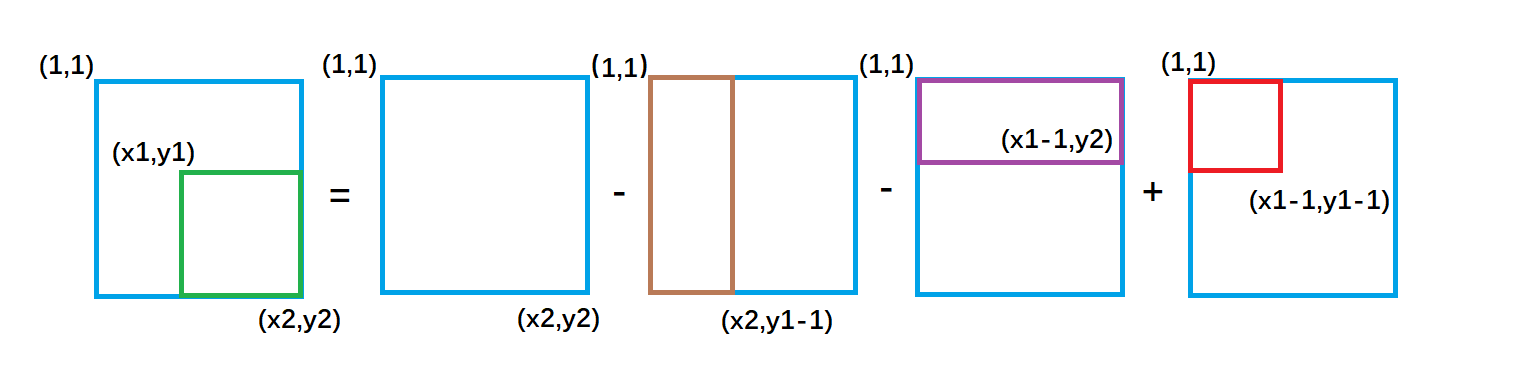绿色矩形的面积 = 整个外围面积s[x2, y2] - 黄色面积s[x2, y1 - 1] - 紫色面积s[x1 - 1, y2] + 重复减去的红色面积 s[x1 - 1, y1 - 1]

因此二维前缀和的结论为：

(x1, y1)为左上角，(x2, y2)为右下角的子矩阵的和为：
s[x2, y2] - s[x1 - 1, y2] - s[x2, y1 - 1] + s[x1 - 1, y1 - 1]

总结：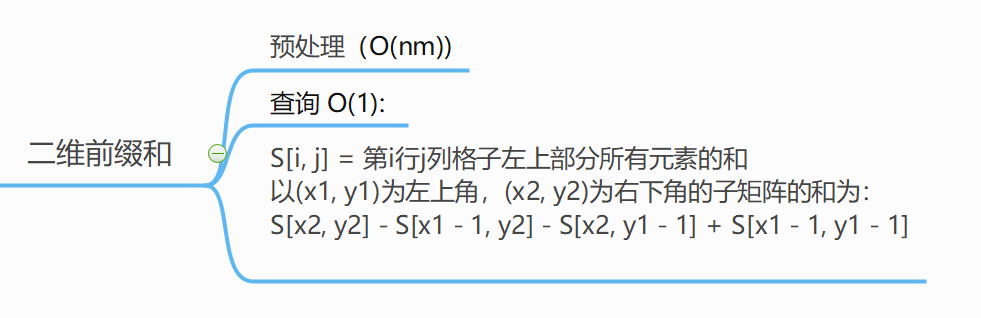练习一道完整题目：
输入一个nm列的整数矩阵，再输入q个询问，每个询问包含四个整数x1, y1, x2, y2，表示一个子矩阵的左上角坐标和右下角坐标。

对于每个询问输出子矩阵中所有数的和。

输入格式
第一行包含三个整数nmq

接下来n行，每行包含m个整数，表示整数矩阵。

接下来q行，每行包含四个整数x1, y1, x2, y2，表示一组询问。

输出格式

q行，每行输出一个询问的结果。

数据范围

1≤n,m≤1000,
1≤q≤200000,
1≤x1≤x2≤n,
1≤y1≤y2≤m,
−1000≤矩阵内元素的值≤1000


输入样例：

3 4 3
1 7 2 4
3 6 2 8
2 1 2 3
1 1 2 2
2 1 3 4
1 3 3 4


输出样例：

17
27
21


代码：

#include <iostream>
using namespace std;
const int N = 1010;
int n, m, q;
int s[N][N];
int main()
{
scanf("%d%d%d", &n, &m, &q);
for (int i = 1; i <= n; i ++ )
for (int j = 1; j <= m; j ++ )
scanf("%d", &s[i][j]);
for (int i = 1; i <= n; i ++ )
for (int j = 1; j <= m; j ++ )
s[i][j] += s[i - 1][j] + s[i][j - 1] - s[i - 1][j - 1];
while (q -- )
{
int x1, y1, x2, y2;
scanf("%d%d%d%d", &x1, &y1, &x2, &y2);
printf("%d\n", s[x2][y2] - s[x1 - 1][y2] - s[x2][y1 - 1] + s[x1 - 1][y1 - 1]);
}
return 0;
}


## 4、差分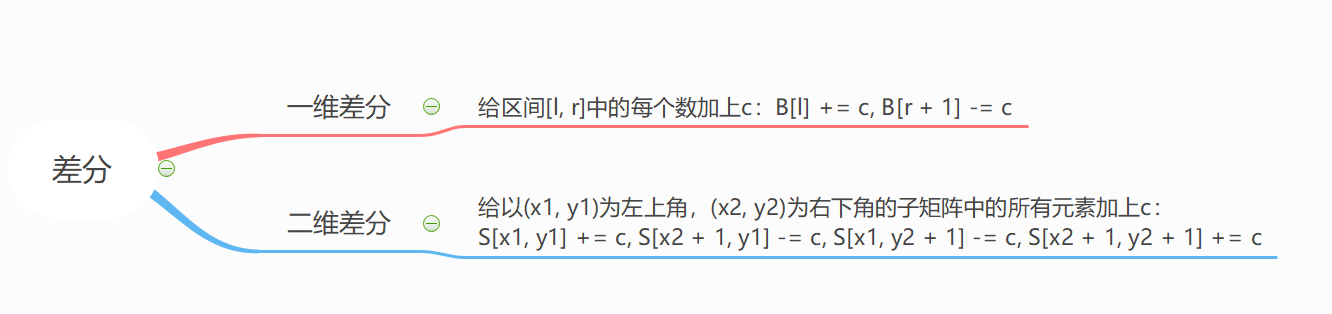## 5、一维差分

类似于数学中的求导和积分，差分可以看成前缀和的逆运算。

差分数组：

首先给定一个原数组aa, a, a,,,,,, a[n];

然后我们构造一个数组bb, b, b,,,,,, b[i];

使得 a[i] = b + b + b + ,,,,,, + b[i]

也就是说，a数组是b数组的前缀和数组，反过来我们把b数组叫做a数组的差分数组。换句话说，每一个a[i]都是b数组中从头开始的一段区间和。

考虑如何构造差分b数组？

最为直接的方法

如下：

a[0 ]= 0;

b = a - a;

b = a - a;

b = a  - a;

........

b[n] = a[n] - a[n - 1];

图示: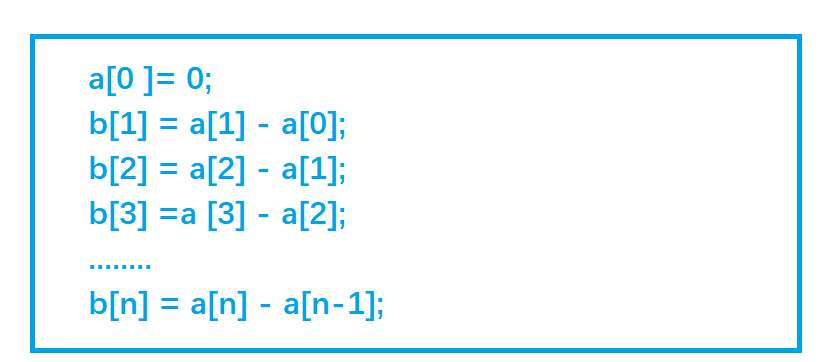我们只要有b数组，通过前缀和运算，就可以在O(n) 的时间内得到 a 数组 。

知道了差分数组有什么用呢？ 别着急，慢慢往下看。

话说有这么一个问题：

给定区间[l, r ]，让我们把a数组中的[l, r] 区间中的每一个数都加上c,即 a[l] + c , a[l + 1] + c , a[l + 2] + c ,,,,,, a[r] + c;

暴力做法是for循环lr区间，时间复杂度O(n)，如果我们需要对原数组执行m次这样的操作，时间复杂度就会变成O(n * m)。有没有更高效的做法吗? 考虑差分做法，(差分数组派上用场了)。

始终要记得，a数组是b数组的前缀和数组，比如对b数组的b[i]的修改，会影响到a数组中从a[i]及往后的每一个数。

首先让差分b数组中的 b[l] + c ,通过前缀和运算，a数组变成  a[l] + c ,a[l + 1] + c,,,,,, a[n] + c;

然后我们打个补丁，b[r + 1] - c, 通过前缀和运算，a数组变成 a[r + 1] - c,a[r + 2] - c,,,,,,,a[n] - c;

为啥还要打个补丁？

我们画个图理解一下这个公式的由来:b[l] + c，效果使得a数组中 a[l] 及以后的数都加上了c(红色部分)，但我们只要求lr 区间加上 c, 因此还需要执行 b[r + 1] - c,让a数组中 a[r + 1]及往后的区间再减去c(绿色部分)，这样对于a[r] 以后区间的数相当于没有发生改变。

因此我们得出一维差分结论：给a数组中的[ l, r] 区间中的每一个数都加上c,只需对差分数组bb[l] + = c, b[r+1] - = c 。时间复杂度为O(1), 大大提高了效率。

总结：题目练习： AcWing 797. 差分

输入一个长度为n的整数序列。
接下来输入m个操作，每个操作包含三个整数l, r, c，表示将序列中[l, r]之间的每个数加上c
请你输出进行完所有操作后的序列。

输入格式
第一行包含两个整数nm
第二行包含n个整数，表示整数序列。
接下来m行，每行包含三个整数lrc，表示一个操作。
输出格式
共一行，包含n个整数，表示最终序列。
数据范围

1≤n,m≤100000,
1≤l≤r≤n,
−1000≤c≤1000,
−1000≤整数序列中元素的值≤1000


输入样例：

6 3
1 2 2 1 2 1
1 3 1
3 5 1
1 6 1


输出样例：

3 4 5 3 4 2


AC代码

#include<iostream>
using namespace std;
const int N = 1e5 + 10;
int a[N],b[N];
int main()
{
int n,m;
scanf("%d%d", &n, &m);
for(int i = 1;i <= n; i++)
{
scanf("%d", &a[i]);
b[i] = a[i] - a[i - 1];      //构建差分数组
}
int l, r, c;
while(m--)
{
scanf("%d%d%d", &l, &r, &c);
b[l] += c;     //表示将序列中[l, r]之间的每个数加上c
b[r + 1] -= c;
}
for(int i = 1;i <= n; i++)
{
b[i] += b[i - 1];  //求前缀和运算
printf("%d ",b[i]);
}
return 0;
}


## 6、二维差分

如果扩展到二维，我们需要让二维数组被选中的子矩阵中的每个元素的值加上c,是否也可以达到O(1)的时间复杂度。答案是可以的，考虑二维差分

a[][]数组是b[][]数组的前缀和数组，那么b[][]a[][]的差分数组

原数组： a[i][j]

我们去构造差分数组： b[i][j]

使得a数组中a[i][j]b数组左上角(1,1)到右下角(i,j)所包围矩形元素的和。

如何构造b数组呢？

其实关于差分数组，我们并不用考虑其构造方法，因为我们使用差分操作在对原数组进行修改的过程中，实际上就可以构造出差分数组。

同一维差分，我们构造二维差分数组目的是为了 让原二维数组a中所选中子矩阵中的每一个元素加上c的操作，可以由O(n*n)的时间复杂度优化成O(1)

已知原数组a中被选中的子矩阵为 以(x1,y1)为左上角，以(x2,y2)为右下角所围成的矩形区域;

始终要记得，a数组是b数组的前缀和数组，比如对b数组的b[i][j]的修改，会影响到a数组中从a[i][j]及往后的每一个数。

假定我们已经构造好了b数组，类比一维差分，我们执行以下操作
来使被选中的子矩阵中的每个元素的值加上c

b[x1][y1] + = c ;

b[x1,][y2+1] - = c;

b[x2+1][y1] - = c;

b[x2+1][y2+1] + = c;

每次对b数组执行以上操作，等价于：

for(int i = x1;i <= x2;i++)
for(int j = y1;j <= y2;j++)
a[i][j] += c;


我们画个图去理解一下这个过程：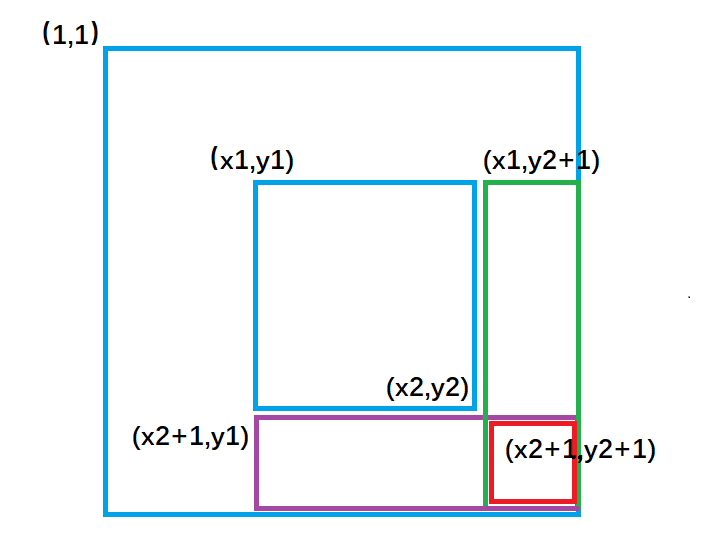b[x1][y1] += c  ; 对应图1 ,让整个a数组中蓝色矩形面积的元素都加上了c
b[x1,][y2 + 1] -= c ; 对应图2 ,让整个a数组中绿色矩形面积的元素再减去c，使其内元素不发生改变。
b[x2 + 1][y1] -= c  ; 对应图3 ,让整个a数组中紫色矩形面积的元素再减去c，使其内元素不发生改变。
b[x2 + 1][y2 + 1] += c; 对应图4,让整个a数组中红色矩形面积的元素再加上c，红色内的相当于被减了两次，再加上一次c，才能使其恢复。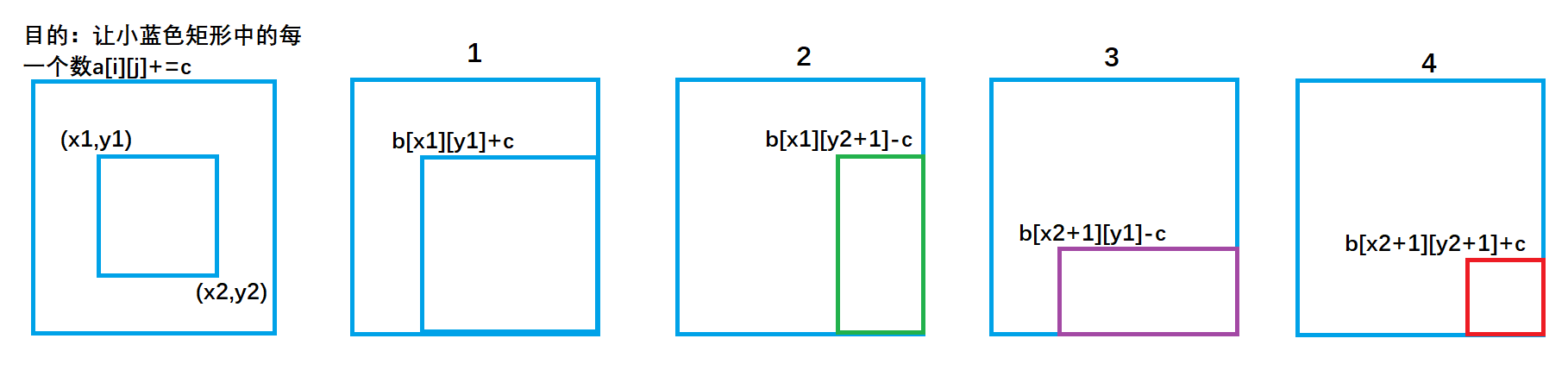我们将上述操作封装成一个插入函数:

void insert(int x1,int y1,int x2,int y2,int c)
{     //对b数组执行插入操作，等价于对a数组中的(x1,y1)到(x2,y2)之间的元素都加上了c
b[x1][y1] += c;
b[x2 + 1][y1] -= c;
b[x1][y2 + 1] -= c;
b[x2 + 1][y2 + 1] += c;
}


我们可以先假想a数组为空，那么b数组一开始也为空，但是实际上a数组并不为空，因此我们每次让以(i,j)为左上角到以(i,j)为右下角面积内元素(其实就是一个小方格的面积)去插入 c = a[i][j] ，等价于原数组a(i,j)(i,j)范围内 加上了 a[i][j] ,因此执行 n*m次插入操作，就成功构建了差分b数组.

这叫做曲线救国。

代码如下：

  for(int i = 1;i <= n;i++)
{
for(int j = 1;j <= m;j++)
{
insert(i, j, i, j, a[i][j]);    //构建差分数组
}
}


当然关于二维差分操作也有直接的构造方法，公式如下：

b[i][j] = a[i][j] − a[i − 1][j] − a[i][j − 1] + a[i −1 ][j − 1]

二维差分数组的构造同一维差分思维相同，因次在这里就不再展开叙述了。

总结：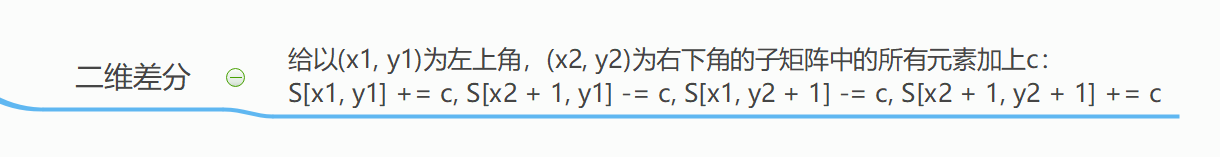题目练习： AcWing 798. 差分矩阵
输入一个nm列的整数矩阵，再输入q个操作，每个操作包含五个整数x1, y1, x2, y2, c，其中(x1, y1)和(x2, y2)表示一个子矩阵的左上角坐标和右下角坐标。
每个操作都要将选中的子矩阵中的每个元素的值加上c
请你将进行完所有操作后的矩阵输出。
输入格式
第一行包含整数n, m, q
接下来n行，每行包含m个整数，表示整数矩阵。
接下来q行，每行包含5个整数x1, y1, x2, y2, c，表示一个操作。
输出格式
n 行，每行 m 个整数，表示所有操作进行完毕后的最终矩阵。
数据范围

1≤n,m≤1000,
1≤q≤100000,
1≤x1≤x2≤n,
1≤y1≤y2≤m,
−1000≤c≤1000,
−1000≤矩阵内元素的值≤1000


输入样例：

3 4 3
1 2 2 1
3 2 2 1
1 1 1 1
1 1 2 2 1
1 3 2 3 2
3 1 3 4 1


输出样例：

2 3 4 1
4 3 4 1
2 2 2 2


AC代码：

#include<iostream>
#include<cstdio>
using namespace std;
const int N = 1e3 + 10;
int a[N][N], b[N][N];
void insert(int x1, int y1, int x2, int y2, int c)
{
b[x1][y1] += c;
b[x2 + 1][y1] -= c;
b[x1][y2 + 1] -= c;
b[x2 + 1][y2 + 1] += c;
}
int main()
{
int n, m, q;
cin >> n >> m >> q;
for (int i = 1; i <= n; i++)
for (int j = 1; j <= m; j++)
cin >> a[i][j];
for (int i = 1; i <= n; i++)
{
for (int j = 1; j <= m; j++)
{
insert(i, j, i, j, a[i][j]);      //构建差分数组
}
}
while (q--)
{
int x1, y1, x2, y2, c;
cin >> x1 >> y1 >> x2 >> y2 >> c;
insert(x1, y1, x2, y2, c);
}
for (int i = 1; i <= n; i++)
{
for (int j = 1; j <= m; j++)
{
b[i][j] += b[i - 1][j] + b[i][j - 1] - b[i - 1][j - 1];  //二维前缀和
}
}
for (int i = 1; i <= n; i++)
{
for (int j = 1; j <= m; j++)
{
printf("%d ", b[i][j]);
}
printf("\n");
}
return 0;
}



如果我的文章对你有帮助，请点点关注，你的关注是对我创作的最大支持。如果你对【前缀和与差分】算法还有疑问的话，欢迎加入我们，一起交流学习！！！展开全文前缀和 差分 前缀和与差分
• ## 前缀和

万次阅读 多人点赞 2019-02-07 10:59:08
对于一个给定的数列 A, 它的前缀和数列S 是通过递推能求出来得 部分和,即数列A中某个下标区间内的数的和,可以表示为前缀和相减的形式: 代码: for(int i = 1...

对于一个给定的数列 A, 它的前缀和数列S 是通过递推能求出来得

部分和,即数列A中某个下标区间内的数的和,可以表示为前缀和相减的形式:

代码:

    for(int i = 1 ; i<=n ; i++ )
cin >>A[i] ;

for(int i = 1 ; i<=n ; i++ )
s[i] = s[i-1] + A[i] ;


所以每次我们询问区间[L,R] 的和,只需要计算s[R] - s[L-1] 就可以了.

[差分]

给你一串长度为n的数列a1,a2,a3......an，要求对a[L]~a[R]进行m次操作：

操作一：将a[L]~a[R]内的元素都加上P

操作二：将a[L]~a[R]内的元素都减去P

最后再给出一个询问求s[L]-s[R]内的元素之和？

当然最暴力的手段是对于每一次操作,我们都要去遍历一下数组再求前缀和,但这样会超时 , 我们用差分就比较方便了.

我们先来直观的认识一下差分数组：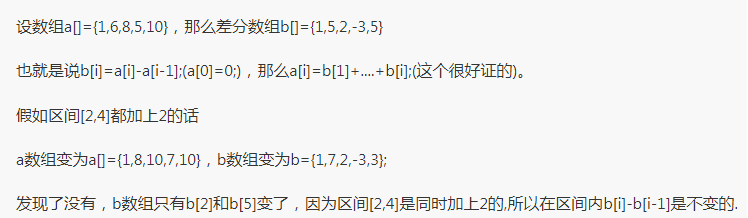### 1 [定义]：

对于已知有n个元素的数列A[1~n],我们可以建立记录它每项与前一项的差值的差分数组B：显然：B=A-0;对于整数i∈[2,n],取A[i]-A[i-1]为其差分数组B[i]的值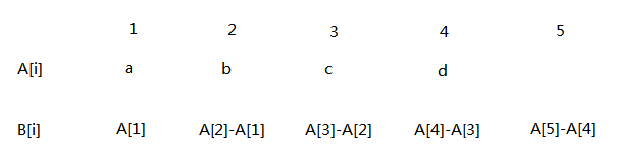### 2 [简单性质]：

(1)计算数列各项的值：可以发现数列A的第i项的值是可以用其差分数组B的前n项和计算，即A[i]=∑B[j](1<=j<=i)

(2)计算数列的前缀和：第i项的前缀和即为数列前i项的和，那么推导可知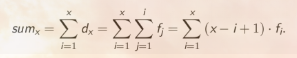即可用差分数组求出数列前缀和

### 3[用途]：

(1)  快速处理区间加减操作：

如果进行区间加减操作，且修改的区间连续，那么若将[x,y]区间内A[i]各加val，我们就可以只对其差分数组B的x和y这两               项进行修改，x项B[x]加val ,y项 B[y+1]减val。

(2)  询问区间和问题：

在保证（1）正确修改的基础上，我们可以由性质（2）计算出数列各项的前缀和数组sum各项的值；那么显然，区间                   [L,R]的和即A[L]+…+A[R] = ans = sum[R]-sum[L-1].

#include<bits/stdc++.h>
using namespace std;
const int maxn=1e5+9;
int a[maxn],b[maxn];
int main(){
int i,j,k,n,m,p;
cin>>n>>m;
for(i=1;i<=n;i++){
cin>>a[i];
}
for(i=1;i<=m;i++){
int L,R,t;
cin>>t>>L>>R>>p;
if(t==1){
b[L]+=p;b[R+1]-=p; //仔细想想为什么b[R+1]要减去p
}
else{
b[L]-=p;b[R+1]+=p;
}
}
for(i=1;i<=n;i++){
}
int x,y;
cin>>x>>y;
cout<<a[y]-a[x-1]<<endl;
}


设a数组表示原始的数组；

设d[i]=a[i]-a[i-1](1<i≤n,d=a)；

设f[i]=f[i-1]+d[i](1<i≤n,f=d=a)；

设sum[i]=sum[i-1]+f[i](1<i≤n,sum=f=d=a)。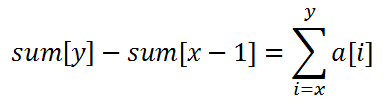举个例子，我们求1~3的区间和.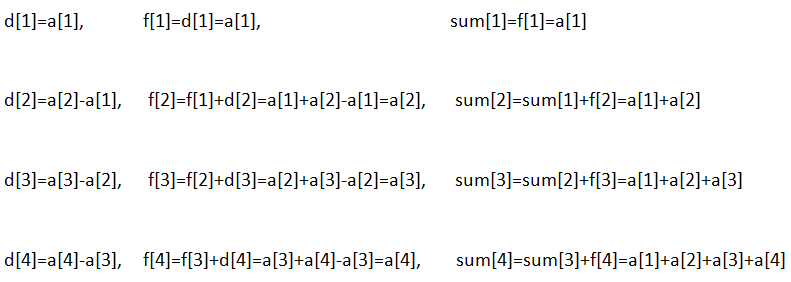后面的可以依次类推。

那么，对于一个操作，我们可以让d[x]加上z，让d[y+1]减小z，就可以了。

还用刚才的例子。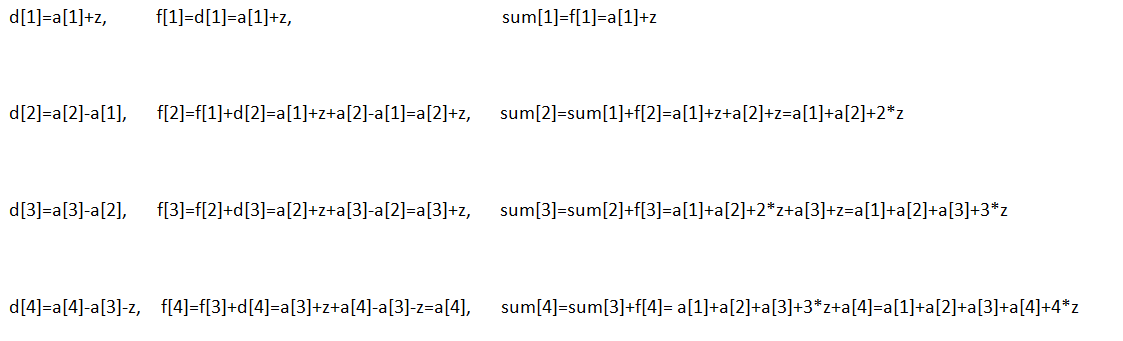后面的可以依次类推。

#include<cstdio>
int n,m,q;
int a,d,f,sum;
int main()
{
int x,y,z;
scanf("%d %d %d",&n,&m,&q);
for(int i=1;i<=n;i++)
{
scanf("%d",&a[i]);
d[i]=a[i]-a[i-1];
}
for(int i=1;i<=m;i++)
{
scanf("%d %d %d",&x,&y,&z);
d[x]+=z;
d[y+1]-=z;
}
for(int i=1;i<=n;i++)
{
f[i]=f[i-1]+d[i];
sum[i]=sum[i-1]+f[i];
}
for(int i=1;i<=q;i++)
{
scanf("%d %d",&x,&y);
printf("%d\n",sum[y]-sum[x-1]);
}
}


对于二维数组前缀和.

如图,我们观察 S[i,j] ,S[i-1,j] , S[i,j-1] , S[i-1,j-1] ;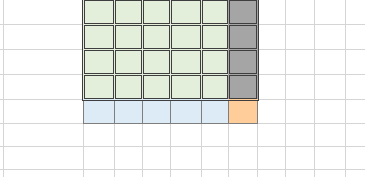S[ i -1 , j ] +S[i , j -1 ]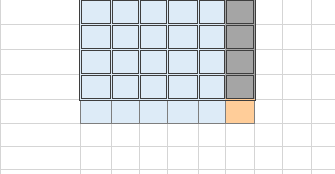S[ i , j-1 ]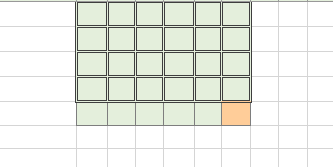S[ i-1,  j ] +S[i , j-1 ] - S[ i- 1 ,j -1 ]

容易得到,  S[ i , j ] = S[ i- 1 ,j ] + S[i , j-1 ]  - S[i - 1 , j-1 ] + A[ i , j ]    , 式子中就包含容斥原理 .

为方便理解贴个图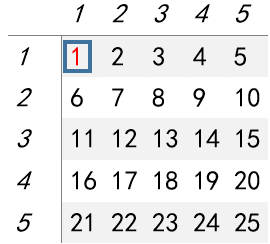假如我想求a的前缀和，我得先加上a的前缀和，再加上a的前缀和，然后这个时候我们发现实际上a这个部分我们加了两遍，所以我们需要再减去一遍a，于是得出公式a[i][j]+=a[i][j-1]+a[i-1][j]-a[i-1][j-1]。

#include<bits/stdc++.h>
using namespace std;
const int maxn=1e3+9;
int a[maxn][maxn];
int main(){
int i,j,k,n,m,q;
cin>>n>>m>>q;
for(i=1;i<=n;i++){
for(j=1;j<=m;j++)
cin>>a[i][j];
}
for(i=1;i<=n;i++){
for(j=1;j<=m;j++)
a[i][j]+=a[i][j-1]+a[i-1][j]-a[i-1][j-1];
}
for(i=1;i<=q;i++){
int x1,y1,x2,y2;
cin>>x1>>y1>>x2>>y2;
int ans=a[x2][y2]-a[x1-1][y2]-a[x2][y1-1]+a[x1-1][y1-1];
cout<<ans<<endl;
}
}


在学完二维前缀和之后，一些同学可能会有疑问，一维前缀和能用上差分，那么二维前缀和能不能用上差分呢？答案是肯定的。

那么怎么差分呢？方法是和一维类似的，我们也是需要另开一个数组记录修改操作，最后求前缀和时统计修改操作，只是二维每一次操作需要记录4个位置，一维只需要记录2个位置。具体怎么做，看下面代码吧。

for(int i=0;i<m;i++){//m是修改操作次数
int x1,y1,x2,y2,p;
cin>>x1>>y1>>x2>>y2>>p;
b[x1][y1]+=p;b[x2+1][y2+1]+=p;
b[x2+1][y1]-=p;b[x1][y2+1]-=p;
}

展开全文• ## python 前缀和总结

千次阅读 2021-06-03 10:53:56
前缀和是数据结构与算法中比较重要的知识，前缀和经常可以结合哈希表解决很多有意思的问题。为了方便学习，在这里总结leetcode中出现的前缀和问题。 525. 连续数组 给定一个二进制数组 nums （只含有0，1）, 找到...

前缀和是数据结构与算法中比较重要的知识，前缀和经常可以结合哈希表解决很多有意思的问题。为了方便学习，在这里总结leetcode中出现的前缀和问题。

525. 连续数组

给定一个二进制数组 nums （只含有0，1）, 找到含有相同数量的 0 和 1 的最长连续子数组，并返回该子数组的长度。

示例 1:

输入: nums = [0,1]
输出: 2
说明: [0, 1] 是具有相同数量0和1的最长连续子数组。
示例 2:

输入: nums = [0,1,0]
输出: 2
说明: [0, 1] (或 [1, 0]) 是具有相同数量0和1的最长连续子数组。

思路：我们用ans来统计数组中0，1出现的次数，即当出现0时，ans - 1，当出现1时，ans + 1。这样我们就可以知道index下出现了0和1出现的个数差。我们用dict来存储ans，key=index，value=ans。因为在统计ans之前，0，1个数差为0，所以初始化时dict = {0：-1}。当ans没有出现在dict时，我们将ans与index记录到dict中，当dict已经存在ans，那么说明这段子序列里0和1出现次数相同，我们计算出子序列的个数，即i - dict[ans]。然后遍历nums找到最长连续子数组的长度。为了方便理解请看下图。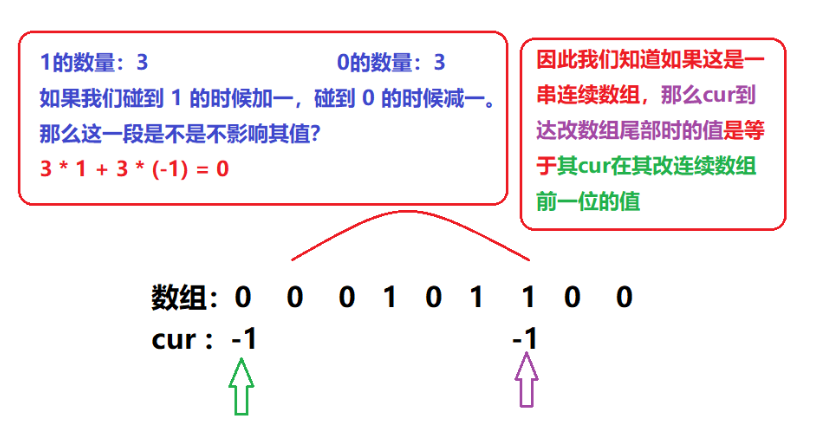class Solution:
def findMaxLength(self, nums: List[int]) -> int:
sum_dict = {0:-1}
ans = 0
res = 0
for i,num in enumerate(nums):
if num == 0:
ans -= 1
elif num == 1:
ans += 1
if ans not in sum_dict:
sum_dict[ans] = i
elif ans in sum_dict:
res = max(res, i - sum_dict[ans])
return res


523. 连续的子数组和
给你一个整数数组 nums 和一个整数 k ，编写一个函数来判断该数组是否含有同时满足下述条件的连续子数组：

子数组大小 至少为 2 ，且
子数组元素总和为 k 的倍数。
如果存在，返回 true ；否则，返回 false 。

如果存在一个整数 n ，令整数 x 符合 x = n * k ，则称 x 是 k 的一个倍数。

示例 1：

输入：nums = [23,2,4,6,7], k = 6
输出：true
解释：[2,4] 是一个大小为 2 的子数组，并且和为 6 。

示例 2：

输入：nums = [23,2,6,4,7], k = 6
输出：true
解释：[23, 2, 6, 4, 7] 是大小为 5 的子数组，并且和为 42 。
42 是 6 的倍数，因为 42 = 7 * 6 且 7 是一个整数。

示例 3：

输入：nums = [23,2,6,4,7], k = 13
输出：false

思路：为了方便计算子数组的和，我们用presum[i]统计0-i索引下的数组和，任意i-j的数组和可以用presum[j] - presum[i]获得。根据同余定理，我们可以用dict存储presum % k的值，当遍历presum时，如果i - dict[presum[i] >=2就返回True

class Solution:
def checkSubarraySum(self, nums: List[int], k: int) -> bool:
presum = *(len(nums) + 1)
for i in range(1,len(presum)):
presum[i] = presum[i-1] + nums[i-1]
presum[i] %= k

predict = {}
for i, re in enumerate(presum):
if re not in predict:
predict[re] = i
for i in range(2, len(presum)):
if i - predict[presum[i]] >= 2:
return True
return False

展开全文数据结构 leetcode 前缀和
• 【一维前缀和】 给定一个数组A[1,2,……n]，则它的前缀和数组为PrefixSum[1..n]。定义为：PrefixSum[i] = A+A+...+A[i-1]； 【例子】 A[5,6,7,8] --> PrefixSum[5,11,18,26] PrefixSum =A ; ...前缀和
• ## 前缀和详解

千次阅读 2021-12-06 08:31:02
目录前缀和概念前缀和代码前缀和例题题目介绍思路分析相关代码总结 前缀和概念 前缀和：顾名思义，是要求前缀的总和，什么是前缀，对于一个存放数字的数组而言，前缀就是指的数组的前k项，因此对应的前缀和就是数组...leetcode java
• ## 前缀和算法

千次阅读 2020-05-10 17:59:10
思路：差分前缀和求出每个数字被查询的次数，然后sort排序，一次赋值n到1，最大的对应n #include<iostream> #include<cstdio> #include<cstring> #include<cmath> #include<algorithm>...前缀和算法
• 什么是前缀和 前缀和（Prefix Sum）的定义为：对于一个给定的数列 A, 它的前缀和数列 S 是通过递推能求出来得 部分和。 例如： C++实现 //假设数组a和前缀和数组s都已经定义 int i; //初始条件 a = 0; s[0...前缀和 一维前缀和
• ## 前缀和算法+实现

千次阅读 2022-03-30 20:06:27
1.前缀和算法 2.前缀和实现 Leetcode 303：区域和检索 - 数组不可变 Leetcode 304：二维区间和检索 - 数组不可变 Leetcode 560：和为K的子数组 3.总结 1.前缀和算法 前缀和的定义：数组从开始至某特定位置...leetcode
• （备注：地区前缀和地区名称也是在VOS客户端导入的数据，在菜单项的号码管理中的地区信息必须要有相应的信息，因为费率表中的地区前缀和地区名称是从地区信息中得到匹配doc文档
• 对于自增（自减）运算，前缀和后缀的优先级有所不同。在重载时候，前缀自增和后缀自增的方法也不相同，本代码详细阐述了两者的区别所在。开发平台VS2010
• 下面我们扩展一下，来介绍二维前缀和。 什么是二维前缀和 比如我们有这样一个矩阵a，如下所示： 1 2 4 3 5 1 2 4 6 3 5 9 我们定义一个矩阵sum，其中，那么这个矩阵就是这样的： 1 3 7 10 6 9 15 22...前缀和 二维前缀和
• 前缀和算法 一维前缀和 即： s=a s=a+a s=a+a+a s=a+a+a+a s=a+a+a+a+a 通过前缀和，我们很容易获取到数组任意 [l ,r]的连续区间的和。后面的前缀和减...java 数据结构
• ## 前缀和（c++实现）

千次阅读 2022-03-30 21:06:39
• 加上前缀和后缀不失为一个好办法下面是收集的一些前缀和后缀，希望能对正在为选择玉米发愁的你提供一点帮助。一 经典后缀(26个)：1 house2 central3 point4 home5 place6 garden7 site8 spot9 park10 dome11 bay12 ...
• 这道题可以简单的先，我们可以用暴力法，但是暴力法只能过70%的数据实际上我们是利用一个前缀和的思想进行求解，只需要求出前缀和，然后得出领域的和，这样我们就不用使用双重循环暴力求解了，具体实现可以看一下...python 前缀和
• ## 前缀和【超详细讲解前缀和】

万次阅读 多人点赞 2020-07-16 17:20:56
对于每个询问，输出原序列中从第l个数到第r个数的。 输入格式 第一行包含两个整数nm。 第二行包含n个整数，表示整数数列。 接下来m行，每行包含两个整数lr，表示一个询问的区间范围。 输出格式 共m行，每行...
• ## 高维前缀和总结

千次阅读 2019-10-09 00:29:31
高维前缀和一般解决这类问题： 对于所有的$$i,0\leq i\leq 2^n-1$$，求解$$\sum_{j\subset i}a_j$$。 显然，这类问题可以直接枚举子集求解，但复杂度为$$O(3^n)$$。如果我们施展高维前缀和的话，复杂度可以到\(O(n...
• ## 基础算法——前缀和详解

千次阅读 多人点赞 2022-03-14 04:10:14
秋名山码民的主页 欢迎关注点赞收藏⭐️留言 作者水平很有限，如果发现错误，一定要及时告知作者 前言 由于有些读者朋友私聊我，希望出...前缀和算法，属于基础算法，一般来说没有固定的模板，但是其思路值得借鉴，我.
• 所谓前缀和可以理解为数学里的数列前n项和，是用来求子区间和的一种便捷方法，与暴力解法相比，其时间复杂度低，大大提高了运行效率。 所谓差分可以理解为前缀和的逆运算，就是将数列中每一项分别与前一项做差。
• 代码： n, m = map(int, input().split()) a = list(map(int, input()... prefix[i + 1] = prefix[i] + a[i] # 求前缀和 # print(prefix) for i in range(m): l, r = map(int, input().split()) print(prefix[r] ..python
• 前面部分我们介绍了一维前缀和...下面我们简单介绍一下树上前缀和。 什么是树上前缀和 假设表示结点 i 到根节点的权值总和。 然后若是点权，路径上的和为； 否...前缀和
• 将中缀表达式，转换为后缀表达式和前缀表达式，再分别计算转换后的表达式值，比较两个计算结果，判断转换正确性计算正确性。 ·编程 （1）读入中缀表达式，表达式的数据可以是实型、整型； （2）转换为后缀表达式...
• ## 五分钟看懂前缀和与差分

千次阅读 多人点赞 2020-05-27 23:01:16
本文主要简单介绍一下前缀和与差分两个内容，主要讲解前缀和与差分的定义，作用和求法三个方面。 因为我们一般认为差分是前缀和的逆运算，所以往往将此两个内容放在一起学习，固此文也按照该模式讲解。 二、前缀和...
• ## c++前缀和与差分

千次阅读 2020-06-04 17:01:11
一、前缀和 1、简介 前缀和也是一个在比赛中比较实用的方法，他能很快得求出一个区间的和，速度为O(1)。 一维的前缀和如sum[ i ]是指数组前i位的所有和，如求区间x到y的和只需sum[ y ] - sum[ x ]。 二维的前缀和如...
• ## C++前缀和

千次阅读 2020-09-24 10:34:38
对于每个询问，输出原序列中从第l个数到第r个数的。 输入格式 第一行包含两个整数nm。 第二行包含n个整数，表示整数数列。 接下来m行，每行包含两个整数lr，表示一个询问的区间范围。 输出格式 共m行，每行......